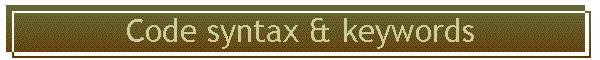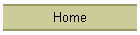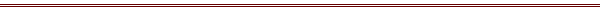C is free form, and it has reserved keywords.  These, and the layout of a typical C routine, are described here.## function return values

In Fortran, there are functions (that return values) and subroutines (that do not).   In C there are only functions, that return results, or void functions, that do not return values.  A void function is the equivalent of a subroutine.

The following is a definition of a routine that returns the square of its argument:
double square( double a)
{
return a*a;
}

The things to notice are the following:  the type of the value returned is declared where the name is declared, "double square.  Its only argument is the variable a, whose type is also declared.  The function returns the product a*a.## header files, and prototypes, for functions

Safe programming practice requires that any routine that uses the "square" defines here, have it  "prototyped" by stating the explicit types of variables to be used.  This allows the compiler to check for any errors in passing different variables to routines, and also "promote" types so that they are correct.  An example of the latter would be if a variable is actually double precision, but the user called it with single precision.  The compiler could generate code to promote the single to double where the call occurred.

A function prototype is very easy to produce.  Just copy the declaration of the function (the part before the open quotes) and place a semicolon after it.  The following would be a declaration of the function given above:

double square( double a);

Normally this line would be put into a file called "square.h", and this file would be included whenever the function was used, and also in the original function itself, by the preprocessors include directive:

#include "square.h"## format

A C line can be continued by simply keeping going.  It ends with a semicolon.   The following will set a to 3.
a
=
3.;
But this will too:
a = 3.;

Carriage returns in the middle of a line will have no effect.  In the case of a character string, the carriage return can be hidden by putting the escape character "\" before it.  The following string will appear as a single line saying "this is a string":
char chString[] =" this is \
a string."## white space counts

Fortran explicitly ignores all white space, so the following lines are equivalent:

do i=1,3
doi = 1,2
d  oi  =  1,   2

The way C defines tokens depends on white space, because C was defined to make the compilers easy to write.  The following statements are not equivalent, and the second will generate an error:

goto label; /* this works, is a goto */
go to label ; /* this is three tokens, go, to, and label, and will generate an error */## main program, its arguments, its return value

In Fortran the main routine can be called anything at all.  In C th main routine must be called main.

The main program receives two arguments from the operating system, conventionally called arc and argv[].  The first is an integer and says how many parameters were present on the command line when the program was executed.  The name of the program is the first parameter, the string in argv.  The following program lists all the parameters it was called with:

#include <stdio.h>
void main( int argc , char argv[] )
{
long i;
for( i=1; i< argc ; ++i )
{
printf(%s\n",arcv)
}
return 0;
}

By convention a successful execution returns the value 0 to the operating system, an non-zero if something bad happened.## statements and blocks

A statement is a single line ending with a semicolon.  There are statements:
a = 0;
long i = 0;
a = c + b;

A block is one or more statements enclosed within a pair of curly brackets, { and }.   A block does not end with a semicolon.  The following if statements first have a statement, then a block, for the true condition:
/* a single statement ending with a semicolon*/
if( a > 0. ) a = log(a) ;
if( b > 0. )
{
a = 1.;
c = 2.;
b = a + c;
}## preprocessor commands

These must start with a # in the first column but can end with almost anything.   Preprocessor commands are commands to a text editor that edits the code before it is sent to the C compiler, and are described here.## reserved keywords

 enum explicit mutable template auto extern naked this bool false namespace thread break float new throw case for operator true catch friend private try char goto protected typedef class if public typeid const inline register typename const_cast int reinterpret_cast union continue long return unsigned default main short using delete signed uuid dllexport sizeof virtual dllimport static void do static_cast volatile double struct wmain dynamic_cast switch while else xalloc## function prototypes

A prototype tells the compiler the types of all variables passed to a routine, and the type of the returned value.  Function prototypes are an important part of safe programming practices, and are required in C++.

To generate a prototype, copy the function definition from its source and add a semicolon after the closing ")".   Place it into a file called the same name as the subroutine but ending in .h, for instance myroutine.h.  Place this prototype anywhere the function is used.

/* this is the declaration of a function */
double MyRoutine( long i )
{
code for function goes here
return value;
}

/* this is the prototype of the function, as used by any routine that want to call it*/
double MyRoutine( long i );## assignment is an operator

Expressions like the following are possible and common:

if( ( a = b+c) == 3 )
{
code goes here
}

The assignment of b+c to a results in the value existing, to be tested against.   This use of an expression inside a logical statement is common, although I consider it bad programming style.  It would be better to do the assignment on one statement, then test the value in a second.

Here is an example of the one place where I use this style, checking on an error condition.  The example declares a file pointer (equivalent to a Fortran IO unit number), tries to open the file, and stops if it was unsuccessful:

/* define the ioUnit we will try to open */
FILE *ioUnit;
/* try to open the file, fopen returns NULL if it didn't work */
if( (ioUnit=fopen("filename.txt", "r" ) )==NULL )
{
code for error condition goes here - the file could not be opened
}